• 编译原理的语法分析树资料资源，考试专用必考必过，985编译原理内部材料编译原理
• 带界面的java实现词法分析器、错误信息、语法分析器、错误信息和语法生成
• C++语言的语法分析器，代码借助yacc和lex,实现了语法书的生成及展示
• 在我的另外两篇文章中介绍了语法分析树建立的Java实现，这次使用flex和bison在Unix环境下完成语法分析树的建立flex介绍bison介绍关于flex和bison的使用和语法在这里就不详细的介绍了。如果你的Unix环境没有装这两个...


在我的另外两篇文章中介绍了语法分析树建立的Java实现，这次使用flex和bison在Unix环境下完成语法分析树的建立flex介绍bison介绍关于flex和bison的使用和语法在这里就不详细的介绍了。如果你的Unix环境没有装这两个工具可以使用如下命令进行安装（机器需要连接网）
sudo apt-get install bison
sudo apt-get install flex按照提示属于“y”即可完成安装

parser.lex

%{
#include "parser.tab.h"
#include "tree.h"
#include <string.h>

int line=1;
int tempTag;
int getTag(char *);

%}

DIGIT 	[0-9]
LETTER	[A-Za-z]
SPACE	\ |\t
LINE	\n
NUM	{DIGIT}+
ID	{LETTER}({LETTER}|{DIGIT})*

%%

{LINE}	{line++;}
{SPACE}	{}

[=]	{yylval=createLeaf(SET,yytext); return SET;}
[!]	{yylval=createLeaf(NOT,yytext); return NOT;}
[-]	{yylval=createLeaf(SUB,yytext); return SUB;}
[*]	{yylval=createLeaf(MUL,yytext); return MUL;}
[/]	{yylval=createLeaf(DIV,yytext); return DIV;}
[>]	{yylval=createLeaf(GT,yytext); return GT;}
[<]	{yylval=createLeaf(LT,yytext); return LT;}
[>][=]	{yylval=createLeaf(GE,yytext); return GE;}
[<][=]	{yylval=createLeaf(LE,yytext); return LE;}
[=][=]	{yylval=createLeaf(EQ,yytext); return EQ;}
[!][=]	{yylval=createLeaf(NE,yytext); return NE;}
[&][&]	{yylval=createLeaf(AND,yytext); return AND;}
[|][|]	{yylval=createLeaf(OR,yytext); return OR;}

[;]	{yylval=createLeaf(SC,yytext); return SC;}
[(]	{yylval=createLeaf(LP,yytext); return LP;}
[)]	{yylval=createLeaf(RP,yytext); return RP;}
[{]	{yylval=createLeaf(LB,yytext); return LB;}
[}]	{yylval=createLeaf(RB,yytext); return RB;}
${yylval=createLeaf(LSB,yytext); return LSB;}$	{yylval=createLeaf(RSB,yytext); return RSB;}

{NUM}	{
yylval=createLeaf(NUM,yytext);
return NUM;
}
{ID}	{
tempTag=getTag(yytext);
yylval=createLeaf(tempTag,yytext);
return tempTag;
}

.	{printf("lexical error: line %d, %s\n", line, yytext);}

%%

int getTag(char *s)
{
if(strcmp(s,"if")==0)
return IF;
else if(strcmp(s,"else")==0)
return ELSE;
else if(strcmp(s,"while")==0)
return WHILE;
else if(strcmp(s,"do")==0)
return DO;
else if(strcmp(s,"break")==0)
return BREAK;
else if(strcmp(s,"true")==0)
return TRUE;
else if(strcmp(s,"false")==0)
return FALSE;
else if(strcmp(s,"int")==0)
return INT;
else if(strcmp(s,"bool")==0)
return BOOL;
else
return ID;
}
parser.y

%{

#include "tree.h"
#include <stdio.h>
#include <stdlib.h>

struct Node *cldArray;
int cldN;
int nTag;

void yyerror (char const *s);
int yylex();

%}

//set attribute type
%define api.value.type {struct Node *}

/* declare tokens */
%token  IF 256  ELSE 257  WHILE 258  DO 259  BREAK 260   TRUE 261  FALSE 262  INT 263  BOOL 264  AND 265  OR 266  NOT 267  EQ 268  NE 269  GT 270  GE 271  LT 272  LE 273  SET 274  ADD 275  SUB 276  MUL 277  DIV 278  SC 279  LP 280  RP 281  LB 282  RB 283  LSB 284  RSB 285  ID 286  NUM 287

%token  EPS 317

%%

program: block {nTag=PRO; cldN=1; cldArray=$1; $$=createNode(nTag, cldN, cldArray); treePrint($$);}; block: LB decls stmts RB {nTag=BLO; cldN=4; cldArray=$1; cldArray=$2; cldArray=$3; cldArray=$4; $$=createNode(nTag, cldN, cldArray);}; decls: {nTag=DECLS; cldN=1; cldArray=createEmpty();$$=createNode(nTag, cldN, cldArray);} | decls decl {nTag=DECLS; cldN=2; cldArray=$1; cldArray=$2; $$=createNode(nTag, cldN, cldArray);}; stmts: {nTag=STMTS; cldN=1; cldArray=createEmpty();$$=createNode(nTag, cldN, cldArray);} | stmts stmt {nTag=STMTS; cldN=2; cldArray=$1; cldArray=$2; $$=createNode(nTag, cldN, cldArray);}; decl: type ID SC {nTag=DECL; cldN=3; cldArray=1; cldArray=2; cldArray=3;$$=createNode(nTag, cldN, cldArray);}; type: type LSB NUM RSB {nTag=TYP; cldN=4; cldArray=$1; cldArray=$2; cldArray=$3; cldArray=$4; $$=createNode(nTag, cldN, cldArray);} | basic {nTag=TYP; cldN=1; cldArray=1;$$=createNode(nTag, cldN, cldArray);}; basic: INT {nTag=BASIC; cldN=1; cldArray=$1; $$=createNode(nTag, cldN, cldArray);} | BOOL {nTag=BASIC; cldN=1; cldArray=1;$$=createNode(nTag, cldN, cldArray);};

stmt: loc SET bexpr SC {nTag=STMT; cldN=4; cldArray=$1; cldArray=$2; cldArray=$3; cldArray=$4; $$=createNode(nTag, cldN, cldArray);} | IF LP bexpr RP stmt {nTag=STMT; cldN=5; cldArray=1; cldArray=2; cldArray=3; cldArray=4; cldArray=5;$$=createNode(nTag, cldN, cldArray);}
| IF LP bexpr RP stmt ELSE stmt {nTag=STMT; cldN=7; cldArray=$1; cldArray=$2; cldArray=$3; cldArray=$4; cldArray=$5; cldArray=$6; cldArray=$7; $$=createNode(nTag, cldN, cldArray);} | WHILE LP bexpr RP stmt {nTag=STMT; cldN=5; cldArray=1; cldArray=2; cldArray=3; cldArray=4; cldArray=5;$$=createNode(nTag, cldN, cldArray);} | DO stmt WHILE LP bexpr RP SC {nTag=STMT; cldN=7; cldArray=$1; cldArray=$2; cldArray=$3; cldArray=$4; cldArray=$5; cldArray=$6; cldArray=$7; $$=createNode(nTag, cldN, cldArray);} | BREAK SC {nTag=STMT; cldN=2; cldArray=1; cldArray=2;$$=createNode(nTag, cldN, cldArray);}
| block {nTag=STMT; cldN=1; cldArray=$1; $$=createNode(nTag, cldN, cldArray);}; loc: loc LSB aexpr RSB {nTag=LOC; cldN=4; cldArray=1; cldArray=2; cldArray=3; cldArray=4;$$=createNode(nTag, cldN, cldArray);} | ID {nTag=LOC; cldN=1; cldArray=$1; $$=createNode(nTag, cldN, cldArray);}; bexpr: bexpr OR join {nTag=BEXP; cldN=3; cldArray=1; cldArray=2; cldArray=3;$$=createNode(nTag, cldN, cldArray);}
| join {nTag=BEXP; cldN=1; cldArray=$1; $$=createNode(nTag, cldN, cldArray);}; join: join AND equality {nTag=JOIN; cldN=3; cldArray=1; cldArray=2; cldArray=3;$$=createNode(nTag, cldN, cldArray);} | equality {nTag=JOIN; cldN=1; cldArray=$1; $$=createNode(nTag, cldN, cldArray);}; equality: equality EQ rel {nTag=EQU; cldN=3; cldArray=1; cldArray=2; cldArray=3;$$=createNode(nTag, cldN, cldArray);}
| equality NE rel {nTag=EQU; cldN=3; cldArray=$1; cldArray=$2; cldArray=$3; $$=createNode(nTag, cldN, cldArray);} | rel {nTag=EQU; cldN=1; cldArray=1;$$=createNode(nTag, cldN, cldArray);}; rel: aexpr LT aexpr {nTag=REL; cldN=3; cldArray=$1; cldArray=$2; cldArray=$3; $$=createNode(nTag, cldN, cldArray);} | aexpr LE aexpr {nTag=REL; cldN=3; cldArray=1; cldArray=2; cldArray=3;$$=createNode(nTag, cldN, cldArray);}
| aexpr GT aexpr {nTag=REL; cldN=3; cldArray=$1; cldArray=$2; cldArray=$3; $$=createNode(nTag, cldN, cldArray);} | aexpr GE aexpr {nTag=REL; cldN=3; cldArray=1; cldArray=2; cldArray=3;$$=createNode(nTag, cldN, cldArray);} | aexpr {nTag=REL; cldN=1; cldArray=$1; $$=createNode(nTag, cldN, cldArray);}; aexpr: aexpr ADD term {nTag=AEXP; cldN=3; cldArray=1; cldArray=2; cldArray=3;$$=createNode(nTag, cldN, cldArray);}
| aexpr SUB term {nTag=AEXP; cldN=3; cldArray=$1; cldArray=$2; cldArray=$3; $$=createNode(nTag, cldN, cldArray);} | term {nTag=AEXP; cldN=1; cldArray=1;$$=createNode(nTag, cldN, cldArray);}; term: term MUL unary {nTag=TERM; cldN=3; cldArray=$1; cldArray=$2; cldArray=$3; $$=createNode(nTag, cldN, cldArray);} | term DIV unary {nTag=TERM; cldN=3; cldArray=1; cldArray=2; cldArray=3;$$=createNode(nTag, cldN, cldArray);}
| unary {nTag=TERM; cldN=1; cldArray=$1; $$=createNode(nTag, cldN, cldArray);}; unary: NOT unary {nTag=UNY; cldN=2; cldArray=1; cldArray=2;$$=createNode(nTag, cldN, cldArray);} | SUB unary {nTag=UNY; cldN=2; cldArray=$1; cldArray=$2; $$=createNode(nTag, cldN, cldArray);} | factor {nTag=UNY; cldN=1; cldArray=1;$$=createNode(nTag, cldN, cldArray);}; factor: LP bexpr RP {nTag=FAC; cldN=3; cldArray=$1; cldArray=$2; cldArray=$3; $$=createNode(nTag, cldN, cldArray);} | loc {nTag=FAC; cldN=1; cldArray=1;$$=createNode(nTag, cldN, cldArray);};
| NUM {nTag=FAC; cldN=1; cldArray=$1; $$=createNode(nTag, cldN, cldArray);}; | TRUE {nTag=FAC; cldN=1; cldArray=1;$$=createNode(nTag, cldN, cldArray);}; | FALSE {nTag=FAC; cldN=1; cldArray=$1; =createNode(nTag, cldN, cldArray);};

%%

int main()
{
yyparse();
return 0;
}

void yyerror (char const *s)
{
fprintf (stderr, "%s\n", s);
}
tree.c

#include "parser.tab.h"
#include "tree.h"
#include <string.h>
#include <stdlib.h>
#include <stdio.h>

struct Node *createLeaf(int tag, char *text)
{
struct Node *nd=(struct Node*)malloc(sizeof(struct Node));
nd->ncld=0;
nd->tag=tag;
if(tag==ID || tag==NUM)
{
nd->value=(char*)malloc(sizeof(char)*strlen(text));
strcpy(nd->value,text);
}
else
nd->value=NULL;
return nd;
}

struct Node *createNode(int tag, int ncld, struct Node *a[])
{
int i;
struct Node *nd=(struct Node*)malloc(sizeof(struct Node));
nd->ncld=ncld;
nd->tag=tag;
nd->value=NULL;
for(i=0; i<nd->ncld; i++)
(nd->cld)[i]=a[i];
return nd;
}

struct Node *createEmpty()
{
struct Node *nd=(struct Node*)malloc(sizeof(struct Node));
nd->ncld=0;
nd->tag=EPS;
nd->value=NULL;

return nd;
}

void treePrintLevel(struct Node *nd, int lvl)
{
int i;
if(nd!=NULL)
{
for(i=0; i<4*lvl; i++)
printf("-");

if(nd->value==NULL)
printf("<%d,->\n", nd->tag);
else
printf("<%d,%s>\n", nd->tag, nd->value);

for (i=0; i<nd->ncld; i++) {
treePrintLevel((nd->cld)[i], lvl+1);
}
}
}

void treePrint(struct Node *nd)
{
treePrintLevel(nd, 0);
}
tree.h

struct Node
{
int tag;
char* value;

struct Node *cld;
int ncld;
};

struct Node *createLeaf(int tag, char *text);
struct Node *createNode(int tag, int ncld, struct Node *a[]);
struct Node *createEmpty();
void treePrint(struct Node * nd);

enum yyNTtype
{
PRO = 300,
BLO = 301,
DECLS = 302,
DECL = 303,
STMTS = 304,
STMT = 305,
TYP = 306,
BASIC = 307,
LOC = 308,
BEXP = 309,
AEXP = 310,
JOIN = 311,
EQU = 312,
REL = 313,
TERM = 314,
UNY = 315,
FAC = 316
};


打开终端输入：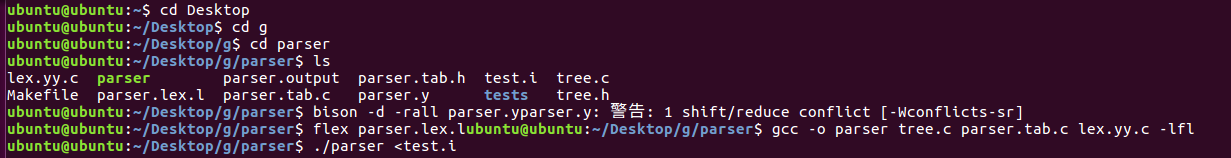运行截图：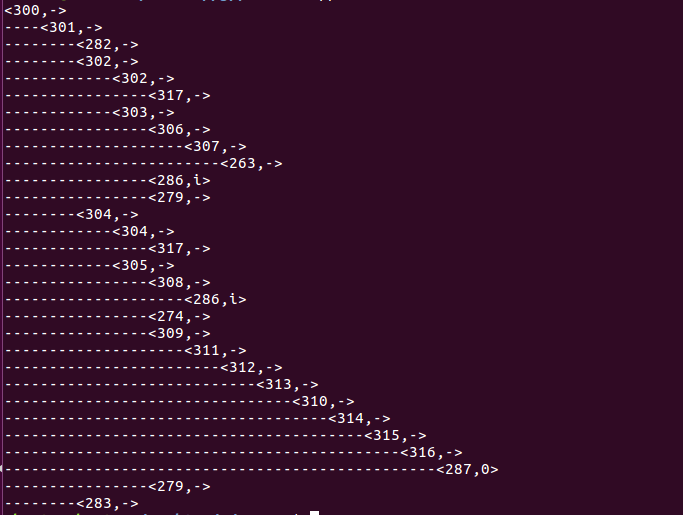parser.lex完成词法分析的工作，parser.y完成语法分析的工作。tree主要是创建树、遍历树的函数的实现，以及数据结构的设计。
展开全文flex bison 编译原理
• 语法分析树是用来描述语法中句子结构的一种树，它能够动态表示一个句子推导的过程。 我们来看一个例子：由左边的文法规则可知，这是一个实现加法减法和乘法的算术表达式。从开始符号E开始，逐步推导，E => -...
语法分析树是用来描述语法中句子结构的一种树，它能够动态表示一个句子推导的过程。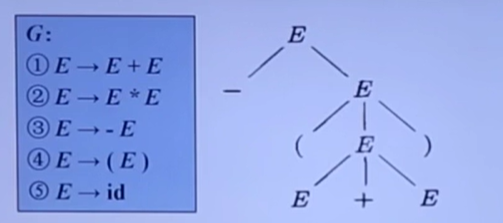我们来看一个例子：由左边的文法规则可知，这是一个实现加法减法和乘法的算术表达式。从开始符号E开始，逐步推导，E => - E,然后 E => (E)等等，最终推导成E => - (E + E).
分析树的根节点对应开始符号。内部节点表示对一个产生式的应用，这个内部节点表示产生式的左部，子节点表示这个产生式的右部。最终的叶子结点则是终结符。

所谓二义性文法：这个文法可以生成多种不同结构的分析树，则这个文法是二义性的。
比如说，某一个文法S和对应的句型：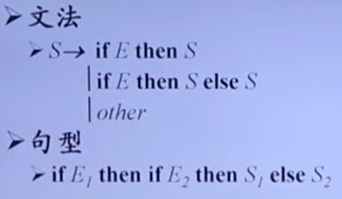那么可以构造出如下两颗分析树：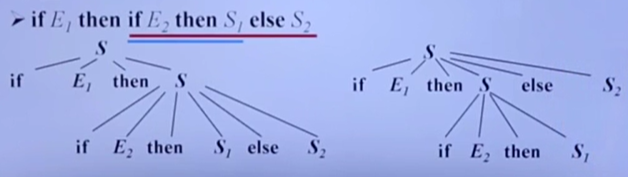其中左边的是红色划线部分构成的，也就是红色划线部分用了文法的第一种形式；而右边的分析树则是文法第二句话构成的。从上面我们能看出，构造出了两种不同的分析树，那么这个文法就是二义性文法。实际上这个二义性是由：有两个if而只有一个else造成的。
对于大部分编译器来说，他们也不希望出现这种歧义。所以解决办法有一种是就近原则，比如上面的else，else最接近哪一个if就认为这个else是在这个if的语法产生式的控制之下，这样就能消除二义性了。


展开全文• 设计一个给定LR分析表，输入一个句子，能由依据LR分析表输出与句子对应的语法树。能对语法树生成过程进行模拟。(算法参见教材)
• 语法分析树用图形方式展现了从文法的开始符号推导出相应语言中的符号串的过程。在具体理解语法分析树之前需要先理清楚一些基本概念：①.产生式用变量expr来表示表达式，用变量stmt表示语句，那么这个构造规则可以...
语法分析树用图形方式展现了从文法的开始符号推导出相应语言中的符号串的过程。在具体理解语法分析树之前需要先理清楚一些基本概念：
①.产生式
用变量expr来表示表达式，用变量stmt表示语句，那么这个构造规则可以表示为：stmt--->if（expr）stmtelse stmt
其中的箭头（--->）可以读作“可以具有以下形式”，这样的规则称为产生式。
②.文法定义关于文法定义中的终结符和非终结符，就参看另外一篇转载的文章。

语法分析树：注释：零个终结符号组成的串称为空串，记为∈。
举例说明： 9 - 5 + 2的语法树分析：根节点的标号为list，即为文法开始的符号。得出文法产生式：
list ---> list + digit
根节点的子节点经过类似推导：
list ---> list - digit
list ---> digit
digit---> 0 | 1 | 2 | 3 | 4 | 5 | 6 | 7 | 8 | 9
一棵语法分析树的叶子节点从左向右构成了树的结果，也就是从根节点上的非终结符号推导得到的符号串：9 - 5 + 2
一句话：为一个给定的终结符号串构建一棵语法分析树的过程称为对该符号串进行语法分析

理解了以上的内容之后，可以尝试做一下龙书第二章的习题，以加深理解：
2.2.1： 考虑下面的上下文无关文法：S → SS+ | SS* |a

1)试说明如何使用该文法生成串aa+a*。

2)试为aa+a*构造一个分析树。

3)该文法产生的语言是什么？

解答：

1)S => SS* => SS+S* => aa+a*

2)3)以a为变量，+和*为二元操作符的后缀表达式

2.2.2 下面的文法产生什么语言？

1)S→0S1|01

{0n1n（n=1,2,…）}

2) S→+SS|-SS|a

以a为变量，+和-为二元操作符的前缀表达式

3)S→S(S)S|ε

括号的匹配

4)S→aSbS|bSaS| ε

由相同数目的a、b组成的字符串，或者空串。

5)S→a|S+S|SS|S*|(S)

以a为数据元素，具有合并、连接、闭包和括号操作符的表达式。a是表达式，若S是表达式则S+S（表达式的合并）、SS（表达式的串联）、S*（表达式的闭包运算）都是表达式。

2.2.3 练习2.2.2中哪些文法具有二义性？

3、4、5具有二义性。

可通过画某个串的分析树来说明


展开全文• 编译原理LR(0)文法分析器 录入合法的LR(0)文法，将输出LR(0)分析表，并可以对输入的句子进行语法分析输出相应语法。程序中部分算法还很不简洁，有待改进，欢迎朋友与我多多交流。 -compiler theory LR (0) grammar...
• 词法分析与语法分析的原始文件扩展： ://www.quut.com/c/ANSI-C-grammar-l-1998.html和 实现了C语言除了struct和指针几乎所有的语法。 运行 环境要求：flex bison g ++ 11 python3 中间代码生成 Windows命令行输入：...
• 使用C++开发一个小型的C语言编译器，实现词法分析，语法分析，语法制导翻译，语义分析和中间代码生成。 数据结构都是使用的C++ STL，语法分析使用的LR(1)分析法。
• java模拟编译器,实现词法,语法分析,生成语法编译器
• 本文介绍了根据上下文无关文法，使用预测分析法生成语法分析树的步骤。

回顾

在开始之前，我们需要知道自己在做什么。  之前，我们花了不少时间熟悉如何将RE转化为DFA，那么为什么要将RE转换为DFA？这个问题类似于现在为什么要将CFG（上下文无关文法）转换成Parser。在掌握具体的步骤之前，我们有必要说明一下这样做的目的。  首先看一下编译器的模型图：为什么要将RE转换到DFA？  相信看过实验一之后这个问题已经非常清楚。实验一我们需要完成一个词法分析器。我们需要自己用正则表达式定义一个语言的成分，比如单词、数字、注释、引用等，分别写出他们对应的NFA，合并NFA并转换成DFA，在得到DFA之后我们可以知道所有的状态和每个状态遇到不同的输入应该如何跳转处理，从DFA生成词法分析的程序。在上一篇文章的一开始，我们也提到了如何从DFA生成程序。词法分析器最终的输出是一个Token序列，记录了lexeme（词）、catalog（种类，就是自己用RE定义的）以及InnnerCode（内部码）  为什么要将CFG转到Parser？  之前我们所做的是词法分析，现在我们需要考虑语法分析的问题，当我们已经定义出一个语言的上下文无关文法，并且拿到了已经分析好的单词序列，我们怎样解析这些单词序列之间的语法关系呢？这类似于如何从有序的单词序列中分析出主语、谓语、宾语。我们需要知道这个单词对应语法中的那种成分，或者这一系列单词究竟符不符合语法要求。
递归下降分析法

有一种很简单的方法可以用来解决这个问题，它叫做递归下降分析法（ Recursive Descent Parsing）。  这个方法利用递归与回搠的方法解决问题，核心在于为每个非终结符构造一个函数。

Example: S=>ABC, A=>aA|ε, B=>bB|ε, C=>cC|ε

首先是main函数：
main() {
i = S();
}
为非终止状态构造函数：
int S() {
i = A();
if (i == 1) {
j = B();
if (j==1) {
return C();
} else {
error();
}
} else {
error();
}
}

int A() {
ch = getChar();
if (ch == 'a') return A();
else return 1;
}
使用这种回搠的方法在面对复杂情况时效率很低，我们需要找出回搠的原因，最期待的情况是经过一定的预处理之后一遍就能判断对。这就需要使用另一种方法：预测分析法。
预测分析法

造成以上方法回搠，主要有两种原因：  - 公共子因子，如A=>aB|aC完全可以变成A=>aE，E=>B|C  - 左递归，如A=>Aa
需要对CFG预处理来消除这两种情况。
1、预处理
1）提取最大左因子（Extract Maximum Common Left Factor）
很简单，就和名字描述的那样，例如A=>aB|aC|bD转换成A=>aE|bD, E=>B|C。
2）消除左递归（Eliminating Left Recursion）
直接左递归
这种情况，只有一种套路。首先来看A=>Aα | β 这种情况，他代表的是以β开头，后面n个α的字符串，因此我们能得到结论：

A=>Aα|β =======> βαn ======> A=>βA’ , A’ => αA’ | ε

有这个结论，我们就可以转换所有左递归的情形。例如：E => E + T | T  把 “+T”看做α，把T看做β，上式就可以改写为 E => TE’ , E’ => +TE’| ε
间接左递归
类似 S=>Qc|c,Q=>Rb|b,R=>Sa|a这种构成了一个环，我们只要把换消除掉就好了，这里可以用到优先级的概念，比如规定S不可以推出Q和R，我们只能将S=Qc|c推倒成S=>Rbc|bc=>Sabc|abc这样就变成了直接递归，用上面的套路就可以解决了。
混合左递归
首先消除直接左递归，然后再消除间接左递归。  就是在出现圈中套圈的情况下要先把内部的圈消掉然后才能消去外部的圈。否则会永远都消不掉，陷入死循环。
2、制表
首先，我们需要再次明确我们的目的：在扫过字符串一遍后就能知道该字符的语法成分。第一步进行的预处理为这样的情况提供了可能。为了达成这一步骤，我们需要知道每个非终止符在遇到特定的字符时应该认定该字符为何种成分。为此，我们需要制定一张表格，描述了非终止符与终止符之间的关系，即如何理解输入的字符序列。
First和Follow都是为了达成上述目标自然形成的概念。  例如，若A=>aB, A=>bC, 那么在状态为A时扫描到a这个字符，我们则知道此时应该将A推导为aB，这个a对应的就是aB中的a。同样，若A=>BC, B=>bC，在遇到b这个字符时，我们仍然知道将A推导为BC，因为A 首先 推导出的终止符是b，这就是First的概念，描述了首先能推导出的终止符的集合。  我们这样定义First：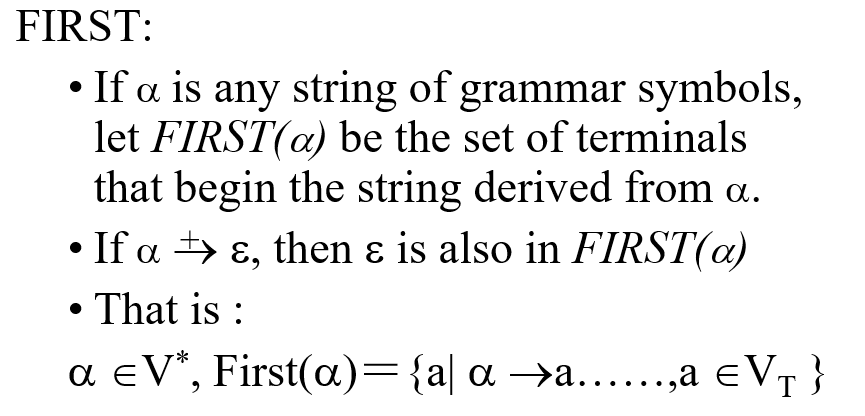若α=>α1α1…αn，则：

First(α) = First(α1) , if ε not in First(α1)  First(α) = (First(α1) - {ε0})∪First(α2) ,if ε∈First(α1)

以此类推，这里提到了ε的情况，若A=>BC, B=>ε|bB, C=>cC，那么A推出的第一个终止符就变成了b，这就是上面定义说明的意思。  可是，若是S=>AD, A=>BC, B=>ε|bB, C=>ε|cC， D=>eD这种情况呢？当我们处于A时，我们对A求First，有b和c和ε，对于这里出现的ε又如何处理呢？当我们处于A，读取的字符是e，我们仍然需要让A推导为BC，让BC都为ε，然后让A后面的D推出eD来对应这个e。所以对于A，我们还需要考虑除了First以外其他有可能在该状态出现的字符。这就要引入Follow的概念。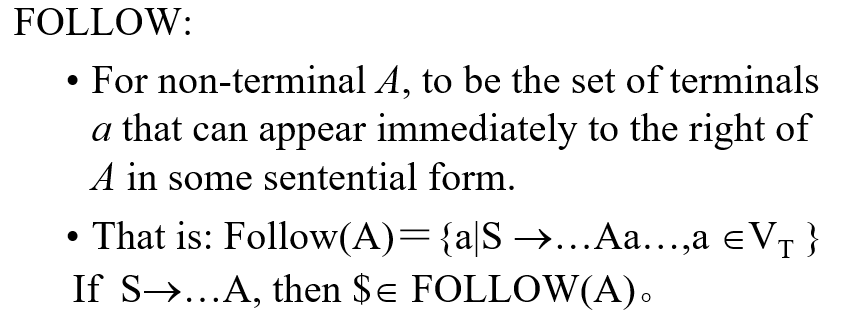即若B=>αAβ，则

Follow(A) = First(β) （β不会推出ε）  Follow(A) = (First(β)-{ε})∪Follow(B) （β推出ε）

若B=>αA，则

Follow(A) <= Follow(B)

但是要是右边什么都没有了呢？如上，我们在最右边加上终止符$，它也可以是Follow的一部分。 最后，我们对经过预处理的CFG的右边求First（如果有ε则求Follow）。 示例：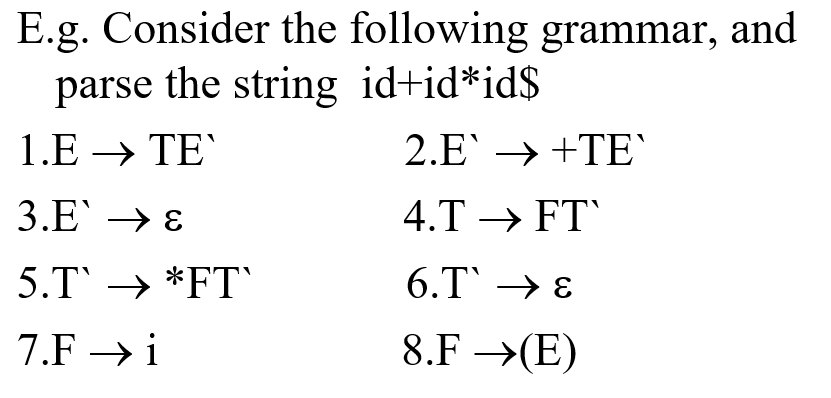可以用上面的方法生成表格：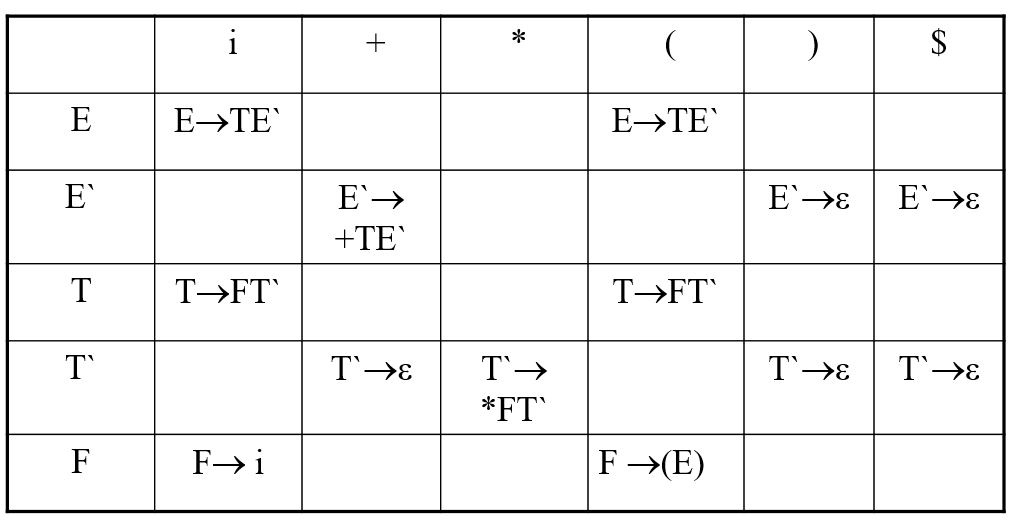比如说，对于E->TE’，我们对TE’求First得到{i,(}，因此i列和（列填上E->TE’。 处理 在获得这张表格之后，剩余的工作就非常简单了。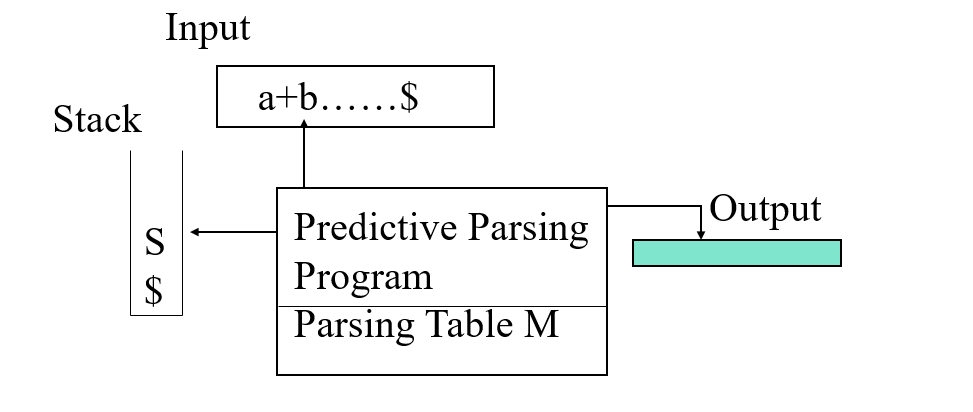首先将$和开始符E压栈（字符序列后需要加上\$），若输入的第一个字符为i，查看上面的表格，E和i对应的是E=>TE’，我们就把E出栈，把E’压栈，把T压栈（注意因为是左推导，左边的需要优先处理，所以左边的要最后压栈），并记下第一步：E=>TE’，重复上面的步骤，若匹配到终止字符，则将输入的字符序列的指针后移，直到匹配到最后的终止符号为止。  之前我们记下每一步的推导式：
E=>TE’T=>FT’F=>iT’=>εE’=>+TE’T=>FT’F=>iT’=>εE’=>ε
根据每一步的推导式可以生成上面的例子中“i+i”的语法分析树：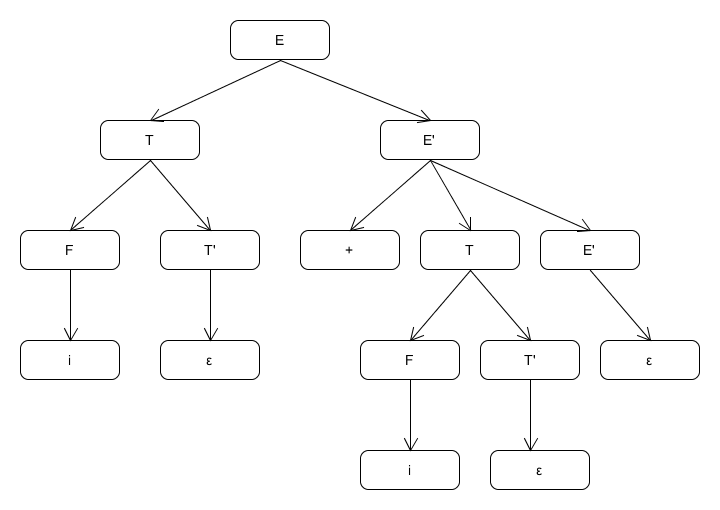LL(1)语法
定义：

A grammar whose parsing table has no multiply-defined entries is said to be LL(1).

LL（1）方法是基于推导的自顶向下的方法。  - The first “L” stands for scanning the input from left to right.从左向右扫描字符串  - The second “L” stands for producing a leftmost derivation.最左推导  - “1” means using one input symbol of look-ahead s.t each step to make parsing action decisions.一步只扫描一个字符
本文的两种方法都只适用于LL（1）语法（在预处理之后符合LL（1））。
展开全文编译原理 上下文无关文法 预测分析法
• ANTLR的运行库提供了两种遍历树的机制：语法分析树监听器与访问器。通过它们，我们可以在遍历树的时候实现相应逻辑。在本章中，我们将通过编写一个简单的计算器来探究三种在事件方法中共享信息的途径。 一、计算器...antlr
• 可分解为：文法输入及解析、分析表构造（含SELECT集求解）、主控程序、语法树展示。 3. 算符优先文法分析器。可分解为：文法输入及解析、分析表构造、主控程序、语法树展示。 4. LR(1)分析器。可分解为：文法输入及...
• LR方法LR parsing是一种相对于LL更通用的方法，LR parser是高效的、自底向上的用于上下文无关文法的语法分析技术。 LR(k)方法中的L、R、K分别代表： L： left-to-right scan从左向右扫描 R：construct a rightmost ...编译原理
• 通过设计、编制、调试一个语法及语义分析原理的理解。LL(1)文法分析过程，构造预测分析C++
• （1）输入任意文法，消除左递归和公共左因子;...　（4）输入一个句子，如果该句子合法则输出与句子对应的语法树； 　能够输出分析过程中每一步符号栈的变化情况。 　如果该句子非法则进行相应的报错处理。
• 如何通过生成java文件的语法分析树来分析源代码，提取源代码中的类名，方法调用，声明结点？有没有之前做过的有相关的代码可以共享一下的？java 源代码
• -这时你需要懂得抽象语法树（AST）。 AST在日常业务中也许很难涉及到，但当你不止于想做一个工程师，而想做工程师的工程师，写出类似webpack，vue-cli前端自动化的工具，或者有批量修改源码的工程需求，那你必须...
• 在学编译原理，自己写的，基于我的另一个词法分析器资源的进一步实现，测试类为src/parser/Test.java，能输出源代码的语法树，希望对你有所帮助
• 编译课里的辅助代码，是你的必备之选，教你如何做一个属于你自己的编译器
• 编译原理语法树的实现~~~~~~~~~~~~~~~~~~~~~~~~~~~~~~~~~~~~~
• 语法分析树中，我们将获得抽象语法树 ，该语法树将用于执行验证并生成已编译的代码。 请注意，术语可能会有所不同：许多人会将从ANTLR获得的树称为抽象语法树。 我更喜欢标记这两个步骤的区别。 对我而言， ...python java 大数据 编程语言 spring
• 数据结构课设 文法分析，生成语法树，用c++编写，可直接使用数据结构 编译原理
• 所有的源码都放GitHub了：https://github.com/yuyi5453/Compilation-principle 成品图： 源码 ...#include"DSJ_词法分析.h" using namespace std; const int max_len=11; char token,token1[40...
• 编译原理实验四，用Flex&Bison;进行语法分析，有正确的.l和.y文件。（实验4 用Yacc工具构造语法分析器）
• c语法分析器，采用bison2.1(yacc), flex(lex), 生成程序的语法 分析单个文件，不支持预处理, 不解析预处理符号# bison，flex工具在上传包内，语法见cgrammar-new.y，词法见input.lex 另附相关说明，本代码采用vs...
• 在文章1.06 使用Flex和Bison手写词法分析器和语法分析器，实现一个简单的计算器中，已经做过类似的事情。但与前一篇文章不同的是，本文将在bison语法分析过程中构建AST，让整个计算器项目更像是一个小型的“编译器”...bison
• 编译原理语法树，编译原理实验，bison，flex...